STUDY
AND
EXAM
.COM

# Preparation for NTS - NAT Test - Guidelines

National Aptitude Test (NAT) is a test designed by NTS for admission in educational institution. NAT test has different types (i.e. NAT-IA, NAT-IM, NAT-IIB etc) depending upon the group of subject for admission.

NAT test has following two major types:

NAT-I  (for student having 12 years of education)
NAT-II (for student having 14 years of education)

Test consists of following four parts:

1. Verbal (English)
2. Quantitative reasoning
3. Analytical reasoning
4. Subject knowledge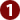## Preparation for Verbal (English)

Verbal part of the test assesses candidates on the basis of their knowledge of grammar and correct usage of vocabulary. The questions consists of:

1. Usage of words e.g. verb, noun, adverb, preposition and conjunction in sentence.
2. Antonyms and synonyms
3. Analogies (word pairs)
4. Paragraph based questions
5. Tense related questions

The guidelines for preparation are as follows:

1. Tenses must be learnt. Some questions are about usage of correct form of verb according to tense. Example: The kid has _______ a cup. (A) break (B) broke (C) broken. Answer: The kid has broken a cup.
2. Some commonly used preposition must be learnt. Two to three questions are always about usage of preposition. Example: They were laughing ____  a Joker. (A) on (B) over (C) after (D) at. Answer: They were laughing at  a Joker.
3. Most of the questions are about synonyms, antonyms and antonyms. Study a good book for English MCQs (e.g. SAT-I). In most of MCQs test these questions are taken from such books.
4. Practice available sample test e.g. from locally available books. Practice helps a lot. You learn much of the content as well as learn test-taking strategies.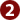## Preparation for Quantitative Part

Quantitative part includes simple arithmetic questions. It is covered from Maths books of class eight, nine and ten. The questions include:

1. Easy arithmetic, e.g. addition, subtraction, division and multiplication.
2. Percentages
3. Ratio and proportion
4. Simple Algebra
5. Simple Geometry e.g. area of shapes and volume of bodies

The guidelines for preparation are as follows:

1. Arithmetic question usually very simple and easy as these are made for all students coming from different educational disciplines.
2. Questions are easy but involve simple tricks.
3. Most of the arithmetic questions have short formulas. Learn these formulas to solve each MCQ quickly. Example: What is sum of all number from 1 to 100? If you start adding all the numbers from 1 to 100, it will take hours. But it has a simple formula.
4. Sum of all digits = total number of digits(first digit + last digit)/2
Sum of all digits = 100 (1 + 100)/2 = 5050 Answer

5. Geometry: Learn the formulas of area of shape and volume of bodies. Each test of many of area and volume questions. Sometime you are asked to find area of an unusual shape. It should not confuse you. Basically such shapes are combination of triangles and squares. You have to find the areas of those triangle and square and add them to find total area of total unusual shape. There may a semi-circle as a part of the shape. So find area of the circle and divide by two.
6. Learn Pythagorean Theorem to find length of one side of triangle. Some questions are based on Pythagorean theorem. You may be asked to find length between two points in a given scenario which is basically a triangle. A commonly used question where length of wall and floor is given. You are asked find the length of ladder to reach to top of wall.
7. Learn the simple basics of calculating percentage as well as ratios.
8. Remember questions are always easy but involve simple tricks. Read the following examples:

Question: If ¾ of a number is 7 more than 1/6 of the number, what is the number?
Options:  A. 12  B. 15    C. 18   D. 20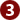## Preparation for Analytical Part

Analytical part assesses your analytical and situation processing skills.

You are given a set of facts and some rules. You have to process the facts according to the given rules.

Examples: Sajid has to study four books (i.e. English, Physics, Chemistry & Biology) on four different day from Thursday to Sunday. He has to study the books according to the following rules:

1. Chemistry on a day before English
2. Biology is studied on a day after Physics

Q. No.1. Which of the following is correct order of books for four days?
A. Chemistry, Biology, English, Physics
B. Biology, Chemistry, English, Physics
C. Physics, Chemistry, Biology, English
D. English, Chemistry, Biology, Physics.

Q. No. 2. If Sajid studies Chemistry on Saturday, which must he have read on Thursday?
A. English
B. Biology
C. Physics
D. Either English or Physics

Q. No. 3. Each of the following is possible EXCEPT:
A. Chemistry on Thursday
B. Physics on Sunday
C. Physics on Thursday
D. Biology on Saturday

Guidelines are as follows:

1. During test, be alert minded and focused on the question.
2. You should have both the facts and rules retained in your mind to solve questions. Usually we just keep the given facts (data) in mind and giving less attention to the rules. You should be focused on both.
3. If the rules statement are too long. Better draw some short symbols on the rough paper to easily grasp them while focusing on the facts during the test.
4. Most of questions are about making combination of items following the given rules. Prepare such questions.
5. For preparation, practice some available tests e.g. from the books. It will help you understand the nature of question and adopt the basics of such question.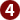## Preparation for Subject Part

The question of subject part is from your concerned subject. Some rules for preparation as follows:

1. Study your subject book with full concentration to learn each topic conceptually.
2. During preparation, have a pencil (or high-lighter) and underline facts and figure in your subject which can be asked in test. It will help you in learning as well as in revising them.
3. Focus on the specific information such as definitions, terminologies, dates, name of scientists and scholars, main assumptions of theories and steps in processes.
4. Be a keen reader and inquisitive learner.
5. Besides your course books, study some MCQs books for your course. It offers you all the MCQs extract from your subject.
6. Revise you books several times.

## Test Taking Strategies

1. MCQs test has very short time. You should divide the total time on number of questions to have an idea of time available for each MCQ.
2. You must have a wrist-watch. Keep an eye on watch during the test to move on timely.
3.  Read every MCQs attentively and speedily.
4. Attempt the easy MCQs first. Leave the difficult MCQs for the end.
5. Don’t stick to one MCQ if it is taking more time. It will waste your time for other easy questions.
6. If any of the MCQs seems complicated to you. First, skip out those options which think cannot be the answer. The remaining options increase chances of selecting the correct option.
7. Practice some tests as home just like appearing in the real test. You will learn the tactics of attempting MCQs test as well as completing the test in time.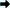Click to attempt 'NTS Practice Test'